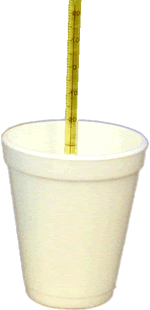# 1.6 Heat & Its Measurement: Calorimetry

All chemical reactions involve energy changes, often in the form of heat. How can you measure the amount of heat exchanged during a reaction?

An instrument called a calorimeter is used. A very simple calorimeter that may be used is shown here. We will use a calorimeter like this one in order to measure the amount of heat exchanged during several simple processes.

In our lab we will dissolve some salts in water. If the reaction is an exothermic reaction, energy is given off, or released, causing the temperature of the water in the calorimeter to rise.

If, however, the reaction is endothermic, energy will be required for the reaction to occur. This energy will be removed from the water, causing the water temperature to drop.

By noting the temperature change of the water in our calorimeter we will be able to calculate how much energy was exchanged.A more sophisticated type of calorimeter is a bomb calorimeter. Research using the Internet to find a diagram of a bomb calorimeter. Better yet, try to locate a movie clip of one being used!
 Quick Research Locate a labelled diagram of a bomb calorimeter on the Internet to include in your notes.

We also need to know the specific heat of water. Specific heat is the amount of energy required to raise the temperature of 1 g of a substance by 1°C.

Water's specific heat is 4.184 J/(g·°C). This means it take 4.184 J of energy to raise the temperature of 1 g of water by 1 °C.
[optional: see note about units]

This value is not a constant, but changes under various conditions. The table to the right lists the specific heats of various substances, including the specific heat of water under a variety of conditions.

Water has a relatively high specific heat which allows it to absorb and release large quantities of heat. This is why temperature changes are often more moderate in areas near large lakes and other large bodies of water. The water absorbs large amounts of heat during hot weather, thus keeping the surrounding area cooler. At night, the water slowly releases heat, thereby warming the air of the surrounding area.

 Specific Heats of various substances at 298 K (25°C) Substance Specific Heat  J/(g·°C) water (liquid) 4.184 water (solid) 2.03 water (steam) 2.01 ethanol (l) 2.44 aluminum (s) 0.897 granite (s) 0.803 iron (s) 0.449 lead (s) 0.129 silver (s) 0.235

### The Calculations

Once we know the mass of water used, the temperature change, and the specific heat of water, the amount of heat transferred by the chemical reaction can now be calculated using the following formula:

 $$\ce{Q= mc \Delta T}$$
where
Q = amount of heat transferred, in kJ
m = mass of the sample in grams
ΔT= change in temperature, in °C
 Lab Activity Heat of Solution Sample data is provided & links to virtual labs

Note - by convention, we indicate an increase in temperature as apositive change and we indicate a decrease in temperature as anegative change. So, if the temperature started at 50°C and ended at 40°C, then ΔT will have a value of -10°C.

Here are some examples of calculating the amount of heat transfer:

Example 1

2,000.0 g of water in calorimeter has its temperature raised 3.0°C by an exothermic chemical reaction. How much heat was transferred?

Solution: (Dimension analysis shows how all units except J will cancel out)

 $$\ce{Q=mc \Delta T}$$ note: since the temperature increased, ΔT will be a positive number

$$\mathrm{=(2000.0g) \times \dfrac{4.184J}{g \cdot ^{ \circ}C} \times 3.0^{ \circ}C}$$

=25,000 J or 25 kJ (to the correct number of significant digits)

There was a net release of 25 kJ of energy released by the reaction.

Example 2

A 1000.0-g mass of water whose temp was 50°C lost 33,600 J of heat over a 5-min period. What was the temperature of the water at the end of the 5-min period?

Solution:

This time, we know the value of Q and are asked to find the final temperature. We will be able to calculate final temperature once we find the change in temperature, ΔT.

Q = mcΔT

You may wish to rearrange the equation first:

$$\mathrm{ \Delta T = \dfrac{Q}{m \times c}}$$

$$\mathrm{ \Delta T = \dfrac{33,600J}{1000.00g \times 4.184 J /(g \cdot ^{\circ}C)} = 8^{ \circ}C}$$

During the reaction, the temperature decreased 8°C. Therefore, the final temperature was 42°C.

$$\mathrm{50 - 8 =42^{ \circ}C}$$